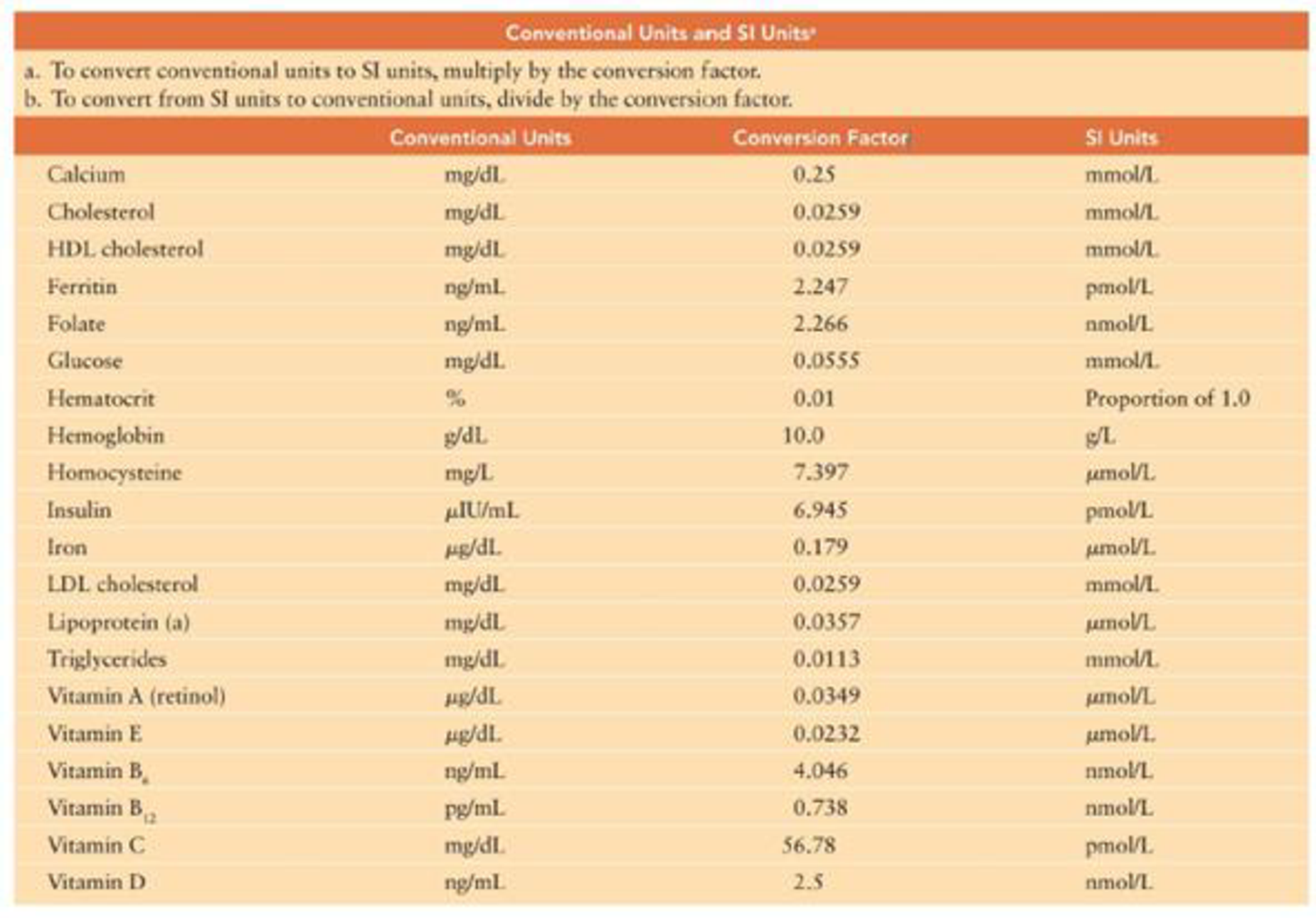Chapter 4, Problem 23RQ

Chapter
Section
Textbook Problem

Use the conversion factors listed in the “Conventional Units to SI Units” table in Appendix A to convert the following conventional unit measures to SI units.52 ng/mL vitamin D (25 hydoxyvitamin D) = ___“SI” stands for le Systeme international d’unites. SI values are based on decades of international cooperation in developing a universal system of measurement.

Summary Introduction

To convert: 52 ng/mL vitamin D (25 hydroxyvitamin D) to its SI units.

Introduction: The International System of Units (SI Units) is defined as a universally agreed system of measurements. They are based upon decades of international cooperation.

Explanation

Refers to the table in Appendix A “Conventional Units to Si Units”, given in the textbook. This table shows that the conventional factor of vitamin D is 2.5.

The conversion of conventional units to SI units is as follows:

SIunit=Conventionalunits×<

Still sussing out bartleby?

Check out a sample textbook solution.

See a sample solution

The Solution to Your Study Problems

Bartleby provides explanations to thousands of textbook problems written by our experts, many with advanced degrees!

Get Started

9.66 Define the term energy density.

Chemistry for Engineering Students

Does the cell cycle refer to mitosis as well as meiosis?

Human Heredity: Principles and Issues (MindTap Course List)

What is the structure and function of a chloroplast?

Biology: The Dynamic Science (MindTap Course List)